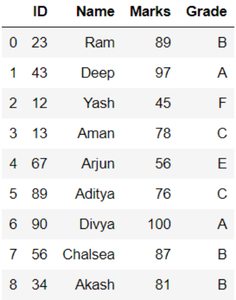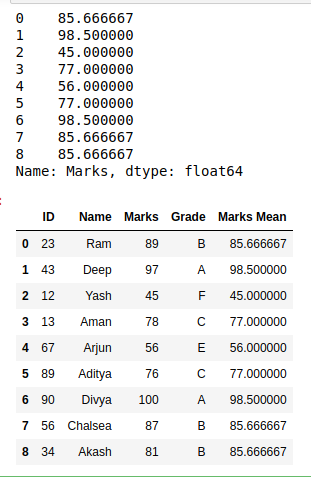# How to Add Group-Level Summary Statistic as a New Column in Pandas?

• Last Updated : 05 Sep, 2020

In this article, we will learn how to Add Group-Level Summary Statistic as a New Column in DataFrame Pandas. This can be done by using the concept of Statistic mean, mode, etc. This requires the following steps :

1. Select a dataframe
2. Form a statistical data from a column or a group of columns
3. Store data as a series
4. Add the series in dataframe as a column.

Here, we take a dataframe, The Dataframe consists of student id, name, marks and grades. Let’s create the dataframe

Attention geek! Strengthen your foundations with the Python Programming Foundation Course and learn the basics.

To begin with, your interview preparations Enhance your Data Structures concepts with the Python DS Course. And to begin with your Machine Learning Journey, join the Machine Learning - Basic Level Course

## Python3

 `# importing packages``import` `pandas as pd`` ` `# dictionary of data``dct ``=` `{``'ID'``: {``0``: ``23``, ``1``: ``43``, ``2``: ``12``,``              ``3``: ``13``, ``4``: ``67``, ``5``: ``89``,``              ``6``: ``90``, ``7``: ``56``, ``8``: ``34``},``        ` `       ``'Name'``: {``0``: ``'Ram'``, ``1``: ``'Deep'``,``                ``2``: ``'Yash'``, ``3``: ``'Aman'``,``                ``4``: ``'Arjun'``, ``5``: ``'Aditya'``,``                ``6``: ``'Divya'``, ``7``: ``'Chalsea'``,``                ``8``: ``'Akash'``},``        ` `       ``'Marks'``: {``0``: ``89``, ``1``: ``97``, ``2``: ``45``, ``3``: ``78``,``                 ``4``: ``56``, ``5``: ``76``, ``6``: ``100``, ``7``: ``87``,``                 ``8``: ``81``},``        ` `       ``'Grade'``: {``0``: ``'B'``, ``1``: ``'A'``, ``2``: ``'F'``, ``3``: ``'C'``,``                 ``4``: ``'E'``, ``5``: ``'C'``, ``6``: ``'A'``, ``7``: ``'B'``,``                 ``8``: ``'B'``}``       ``}`` ` `# create dataframe``df ``=` `pd.DataFrame(dct)`` ` `# view dataframe``df`

Output:Now, we will find the group level statistics summary using the above approach.

## Python3

 `# make a series``new_column ``=` `df.groupby(``'Grade'``).Marks.transform(``'mean'``)`` ` `# view new series``print``(new_column)`` ` `# add column in dataframe``df[``"Marks Mean"``] ``=` `df.groupby(``'Grade'``).Marks.transform(``'mean'``)`` ` `# view modified dataframe``print``(df)`

Output:My Personal Notes arrow_drop_up